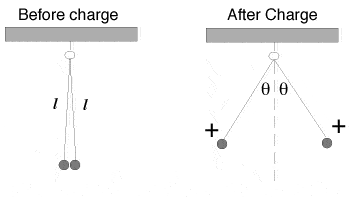# Having trouble with a simple problem

Two insulated balls of mass 0.1 g hang from the same support point by massless insulating threads of length l (as shown in the diagram below.) A total positive charge of 5.70 x 10-07 C is added to the system. Half this charge is taken up by each ball, distributed uniformly, and the balls spread apart to a new equilibrium position.Assuming that the balls hang essentially vertical before the charge is added, what is the tension in each thread before the charge is added?

T=-m*g

I believe that since the strings are mass less and said to be essentially vertical, then the only equation i should need is Tension=-mass*gravity to get the force of tension on each string. so I have T=-(.1 g)(-9.8 m/s2)=.98 g*m/s2=.98 N but when I enter it onto my course site it tells me I am incorrect. Am i being oblivious to something?

I notice that you multiply 2 negatives in the last line, should not one of these be +ve?

I notice that you multiply 2 negatives in the last line, should not one of these be +ve?

In my problem i am orienting the gravity to go in a negative direction, thus force on the ball is F=(.1 g)(-9,8 m/s2)=-.98 N, however, the tension in the rope acting on the ball is in the opposite direction (if i am wrong, please explain), so the Tension will be a positive value.

btw. I tried putting in -.98 N as well on the site and it told me i was incorrect. This is driving me crazy, it seems so simple!

Are you including the Coulombic force?

btw. I tried putting in -.98 N as well on the site and it told me i was incorrect. This is driving me crazy, it seems so simple!

The balls are 0.1 g, not 0.1 kg